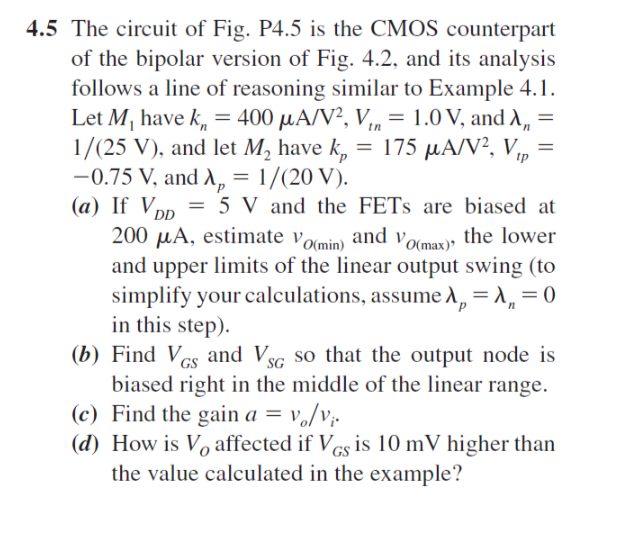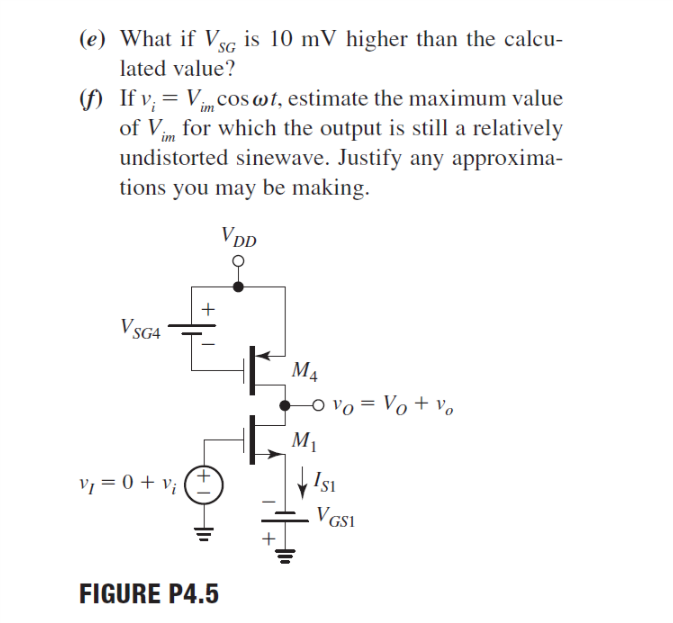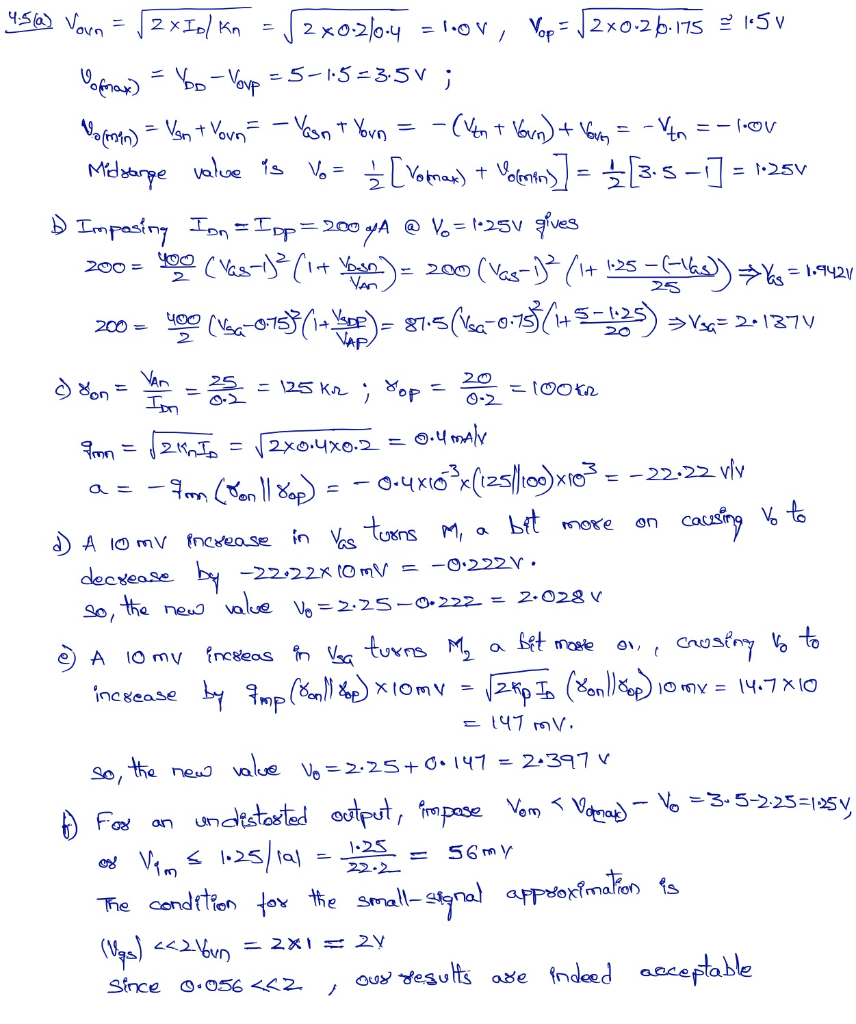# 4.5 The circuit of Fig. P4.5 is the CMOS counterpart of the bipolar version of Fig. 4.2, and its ...4.5 The circuit of Fig. P4.5 is the CMOS counterpart of the bipolar version of Fig. 4.2, and its analysis follows a line of reasoning similar to Example 4.1. Let Mi have kn 400 μΑΛ72, V,,-1 .0 V, and λ,- 1/(25 V), and let M. have kp = 175 μΑ/V, V,,- -0.75 V. and λ,-1/(20 V). (a) If VpD5 V and the FETs are biased at 200 μΑ, estimate vomin) and vo(max), t and upper limits of the linear output swing (to simplify your calculations, assume λ,-X,-0 х» in this step) (b) Find Vs and Vsc so that the output node is biased right in the middle of the linear range. (c) Find the gain a -Vo/v (d) llow is V,, illecied il·Yu is lO1nV hishicrihan the value calculated in the example?
(e) What if Vsc is 10 mV higher than the calcu- lated value? If vi-Vim cos ω. estimate the maximum value of V for which the output is stll a relatively undistorted sinewave. Justify any approxima- tions vou may be making. V. SG4 4 S1 GSI FIGURE P4.5##### Add Answer of: 4.5 The circuit of Fig. P4.5 is the CMOS counterpart of the bipolar version of Fig. 4.2, and its ...
More Homework Help Questions Additional questions in this topic.

• #### So with almost no impact on its sales and little concern amont its customers, should Wal-mart take on its critics and ight back, or shoul it focus on its business and let its results speak for themselves

Need Online Homework Help?